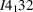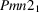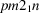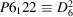International Tables for Crystallography (2015). Vol. A. ch. 1.4, pp. 42-73https://doi.org/10.1107/97809553602060000922

## Contents

• 1.4. Space groups and their descriptions  (pp. 42-74)
• 1.4.1. Symbols of space groups  (pp. 42-50) | html | pdf |
• 1.4.1.1. Introduction  (p. 42) | html | pdf |
• 1.4.1.2. Space-group numbers  (p. 42) | html | pdf |
• 1.4.1.3. Schoenflies symbols  (pp. 42-43) | html | pdf |
• 1.4.1.3.1. Schoenflies symbols of the crystal classes  (pp. 42-43) | html | pdf |
• 1.4.1.3.2. Schoenflies symbols of the space groups  (p. 43) | html | pdf |
• 1.4.1.4. Hermann–Mauguin symbols of the space groups  (pp. 43-48) | html | pdf |
• 1.4.1.4.1. Introduction  (pp. 43-44) | html | pdf |
• 1.4.1.4.2. General aspects  (pp. 44-45) | html | pdf |
• 1.4.1.4.3. Triclinic space groups  (pp. 45-46) | html | pdf |
• 1.4.1.4.4. Monoclinic space groups  (p. 46) | html | pdf |
• 1.4.1.4.5. Orthorhombic space groups  (pp. 46-47) | html | pdf |
• 1.4.1.4.6. Tetragonal space groups  (p. 47) | html | pdf |
• 1.4.1.4.6.1. Tetragonal space groups with one symmetry direction  (p. 47) | html | pdf |
• 1.4.1.4.6.2. Tetragonal space groups with three symmetry directions  (p. 47) | html | pdf |
• 1.4.1.4.7. Trigonal, hexagonal and rhombohedral space groups  (pp. 47-48) | html | pdf |
• 1.4.1.4.7.1. Trigonal space groups  (pp. 47-48) | html | pdf |
• 1.4.1.4.7.2. Hexagonal space groups  (p. 48) | html | pdf |
• 1.4.1.4.7.3. Rhombohedral space groups  (p. 48) | html | pdf |
• 1.4.1.4.8. Cubic space groups  (p. 48) | html | pdf |
• 1.4.1.5. Hermann–Mauguin symbols of the plane groups  (pp. 48-49) | html | pdf |
• 1.4.1.6. Sequence of space-group types  (pp. 49-50) | html | pdf |
• 1.4.2. Descriptions of space-group symmetry operations  (pp. 50-59) | html | pdf |
• 1.4.2.1. Symbols for symmetry operations  (pp. 50-51) | html | pdf |
• 1.4.2.2. Seitz symbols of symmetry operations  (pp. 51-53) | html | pdf |
• 1.4.2.3. Symmetry operations and the general position  (pp. 53-55) | html | pdf |
• 1.4.2.4. Additional symmetry operations and symmetry elements  (pp. 55-56) | html | pdf |
• 1.4.2.5. Space-group diagrams  (pp. 56-59) | html | pdf |
• 1.4.3. Generation of space groups  (pp. 59-61) | html | pdf |
• 1.4.3.1. Selected order for non-translational generators  (pp. 60-61) | html | pdf |
• 1.4.4. General and special Wyckoff positions  (pp. 61-67) | html | pdf |
• 1.4.4.1. Crystallographic orbits  (pp. 61-62) | html | pdf |
• 1.4.4.2. Wyckoff positions  (pp. 62-64) | html | pdf |
• 1.4.4.3. Wyckoff sets  (pp. 64-66) | html | pdf |
• 1.4.4.4. Eigensymmetry groups and non-characteristic orbits  (pp. 66-67) | html | pdf |
• 1.4.5. Sections and projections of space groups  (pp. 67-73) | html | pdf |
• 1.4.5.1. Introduction  (pp. 67-68) | html | pdf |
• 1.4.5.2. Sections  (pp. 68-71) | html | pdf |
• 1.4.5.3. Projections  (pp. 71-73) | html | pdf |
• References | html | pdf |
• Figures
• Fig. 1.4.1.1. Symmetry-element diagrams of some point groups  (p. 42) | html | pdf |
• Fig. 1.4.2.1. General-position and symmetry-operations blocks for the space group, No. 14 (unique axis b, cell choice 1)  (p. 50) | html | pdf |
• Fig. 1.4.2.2. General-position and symmetry-operations blocks as given in the space-group tables for space group Fmm2 (42)  (p. 51) | html | pdf |
• Fig. 1.4.2.3. Symmetry-element diagram for space group Fmm2 (42) (orthogonal projection along )  (p. 56) | html | pdf |
• Fig. 1.4.2.4. General-position and symmetry-operations blocks as given in the space-group tables for space group P4mm (99)  (p. 56) | html | pdf |
• Fig. 1.4.2.5. Symmetry-element diagram for space group P4mm (99) (orthogonal projection along )  (p. 56) | html | pdf |
• Fig. 1.4.2.6. Symmetry-element diagram (left) and general-position diagram (right) for the space group, No. 14 (unique axis b, cell choice 1)  (p. 57) | html | pdf |
• Fig. 1.4.2.7. Symmetry-element diagram (left) and general-position diagram (right) for the space group P2, No. 3 (unique axis b, cell choice 1)  (p. 58) | html | pdf |
• Fig. 1.4.2.8. General-position diagrams for the space group(214)  (p. 58) | html | pdf |
• Fig. 1.4.4.1. General-position block as given in the space-group tables for space group P4bm (100)  (p. 63) | html | pdf |
• Fig. 1.4.4.2. Symmetry-element diagram for the space group P4bm (100) for the orthogonal projection along   (p. 64) | html | pdf |
• Fig. 1.4.4.3. Symmetry-element diagram for the space group P4 (75) for the orthogonal projection along   (p. 65) | html | pdf |
• Fig. 1.4.4.4. Symmetry-element diagrams for the space group(17) for orthogonal projections along , ,  (left to right)  (p. 65) | html | pdf |
• Fig. 1.4.5.1. Duality between section and projection  (p. 68) | html | pdf |
• Fig. 1.4.5.2. Symmetry-element diagrams for the space group(31) for orthogonal projections along  (left),  (middle) and  (right)  (p. 70) | html | pdf |
• Fig. 1.4.5.3. Symmetry-element diagram for the layer group(32)  (p. 70) | html | pdf |
• Fig. 1.4.5.4. Orthogonal projection along  of the symmetry-element diagram for(31) (top) and the diagram for plane group p2mg (7) (bottom)  (p. 72) | html | pdf |
• Fig. 1.4.5.5. `Symmetry of special projections' block of(117) as given in the space-group tables  (p. 73) | html | pdf |
• Fig. 1.4.5.6. Orthogonal projection along  of the symmetry-element diagram for(117) (left) and the diagram for plane group p4gm (12) (right)  (p. 73) | html | pdf |
• Tables
• Table 1.4.1.1. The structure of the Hermann–Mauguin symbols for the space groups  (p. 45) | html | pdf |
• Table 1.4.1.2. The structure of the Hermann–Mauguin symbols for the plane groups  (p. 48) | html | pdf |
• Table 1.4.1.3. List of geometric crystal classes  (p. 49) | html | pdf |
• Table 1.4.2.1. Linear parts R of the Seitz symbolsfor space-group symmetry operations of cubic, tetragonal, orthorhombic, monoclinic and triclinic crystal systems  (p. 52) | html | pdf |
• Table 1.4.2.2. Linear parts R of the Seitz symbolsfor space-group symmetry operations of hexagonal and trigonal crystal systems  (p. 52) | html | pdf |
• Table 1.4.2.3. Linear parts R of the Seitz symbolsfor symmetry operations of rhombohedral space groups (rhombohedral-axes setting)  (p. 52) | html | pdf |
• Table 1.4.2.4. Linear parts R of the Seitz symbolsfor plane-group symmetry operations of oblique, rectangular and square crystal systems  (p. 53) | html | pdf |
• Table 1.4.2.5. Linear parts R of the Seitz symbolsfor plane-group symmetry operations of the hexagonal crystal system  (p. 53) | html | pdf |
• Table 1.4.2.6. Right coset decomposition of space group, No. 14 (unique axis b, cell choice 1) with respect to the normal subgroup of translations(p. 54) | html | pdf |
• Table 1.4.3.1. Sequence of generators for the crystal classes  (p. 59) | html | pdf |
• Table 1.4.3.2. Generation of the space group(178)  (p. 60) | html | pdf |
• Table 1.4.5.1. Coset representatives of(31) relative to its translation subgroup  (p. 69) | html | pdf |
• Table 1.4.5.2. Coset representatives of(117) relative to its translation subgroup  (p. 73) | html | pdf |